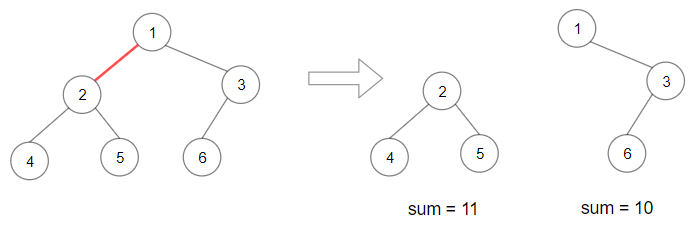# Leetcode Weekly Contest 174 题解

## Leetcode 1341. The K Weakest Rows in a Matrix

Given a m * n matrix mat of ones (representing soldiers) and zeros (representing civilians), return the indexes of the k weakest rows in the matrix ordered from the weakest to the strongest.
A row i is weaker than row j, if the number of soldiers in row i is less than the number of soldiers in row j, or they have the same number of soldiers but i is less than j. Soldiers are always stand in the frontier of a row, that is, always ones may appear first and then zeros.

Example 1:

## 1342. Reduce Array Size to The Half

Given an array arr. You can choose a set of integers and remove all the occurrences of these integers in the array.
Return the minimum size of the set so that at least half of the integers of the array are removed.

### 1343. Maximum Product of Splitted Binary Tree

Given a binary tree root. Split the binary tree into two subtrees by removing 1 edge such that the product of the sums of the subtrees are maximized.
Since the answer may be too large, return it modulo 10^9 + 7.

Example 1:BTW 这段代码的 type hint 使用其实有点问题，我后面比赛完了改了一版

BTW，这道题因为数据太大，需要对 10^9+7 取模，我智障的忘了取模，WA 了两次，罚时罚哭。。。我真的太菜了。。

## 1344. Jump Game V

Given an array of integers arr and an integer d. In one step you can jump from index i to index:
i + x where: i + x < arr.length and 0 < x <= d.
i - x where: i - x >= 0 and 0 < x <= d.
In addition, you can only jump from index i to index j if arr[i] > arr[j] and arr[i] > arr[k] for all indices k between i and j (More formally min(i, j) < k < max(i, j)).
You can choose any index of the array and start jumping. Return the maximum number of indices you can visit.
Notice that you can not jump outside of the array at any time.1. i+x < arr.length 和 0<x<=d

2. i-x >=0 和 0<x<=d